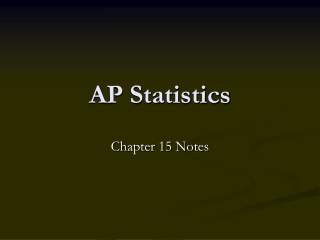DownloadDownload PresentationAP Statistics

# AP Statistics

Télécharger la présentation## AP Statistics

- - - - - - - - - - - - - - - - - - - - - - - - - - - E N D - - - - - - - - - - - - - - - - - - - - - - - - - - -
##### Presentation Transcript

1. AP Statistics Chapter 15 Notes

2. Inference for a Regression Line • Goal: To determine if there is a relationship between two quantitative variables. • i.e. What is the slope of the regression line? • From sample…a and b are statistics • True regression line…α and β are parameters.

3. Conditions • 1. Observations are independent. • 2. True relationship is linear. • 3. σ is constant. • Check residual plot…make sure there is no pattern and the magnitude of the residuals is stable. • 4. Response varies Normally about the true regression line. • Make histogram or stemplot of residuals.

4. Confidence Interval (estimate β) • b ± t* SEb (df = n – 2) • Significance Test • Ho: β = 0 t = b/SEb • Ha: β ≠ > < 0